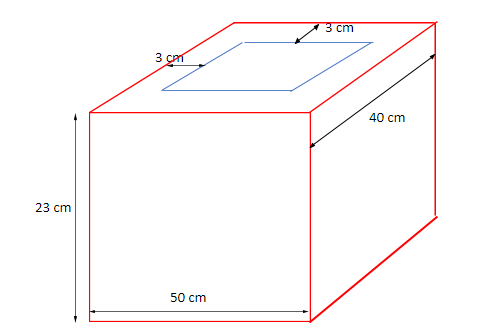The dimensions of an open box are 50 cm, 40 cm and 23 cm. Its thickness is 3 cm. If 1 cubic cm of metal used in the box weighs 0.5 gms, find the weight of the box.6.2 kg12kg7.99 kg8.04 kgVerified
145.8k+ views
Hint: Here, we are given the dimensions of an open box. So firstly, we will calculate the volume of the box using the formula: outer volume – inner volume and it will be equal to the amount of metal used. Then we are given the weight of metal per cubic cm. so, we can calculate the total weight of the open box by multiplying this weight with the volume of the box.

First of all, we are given the dimensions of an open box as 50 cm, 40 cm and 23cm. We are also provided the thickness of the box which is 3 cm.
Let us draw the figure of the given situation.We can observe from the figure that there is an opening on top of the box.
Now, we will calculate the volume of this given box. As we can see, we need to subtract the inner volume of the given box from the outer volume of the box.
For that, let us look at the dimensions of outerbox and inner box.
For the outer box, dimensions will be L: 50 cm
B: 40 cm
H: 23 cm
And for the inner box, dimensions will get reduced by 3 cm due to the thickness of the box. We will subtract 6 cm from the length and width of it because if we look at any side of the box, it will have thickness on either end of it (3cm $\times$2). We will subtract only 3 cms from height because the given box is open from the top, so only base thickness will be excluded.
Therefore, the dimensions of the inner box are: L: 50 – 6 = 44 cm
B: 40 – 6 = 34 cm
H: 23 – 3 = 20 cm
So, the volume of the box = volume of the outer box – volume of the inner box
$\Rightarrow$volume of the box = $\left( {50 \times 40 \times 23} \right) - \left( {44 \times 34 \times 20} \right)$ cm3
$\Rightarrow$volume of the box = 46000 – 29920 ${cm}^{3}$
$\Rightarrow$volume of the box = 16080 ${cm}^{3}$
Now, we need to find the weight of the box. Weight of the box is equal to the product of its weight per cubic cm and total amount of metal used.
We know that here weight per cubic cm is given as 0.5 gms and also that the amount of metal used will be equal to the volume of the box.
Therefore, forming an equation and putting the values into it, we get
Weight of the box = weight of the box per cubic cm $\times$ total amount of metal used
$\Rightarrow$ weight of the box = 0.5 $\times$ 16080 = 8040 gms.
Therefore, we found that the weight of the given open box is 8040 gms. We can write 8040 gms as 8.040 kg as 1 kg = 1000gms.

Hence, option(D) is correct.

Note: In such problems, you can go wrong while considering the dimensions of the metallic box when the condition that the box is open is provided. You need to take care of calculations and conversions as well.